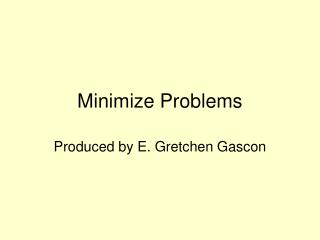DownloadDownload PresentationMinimize Problems

# Minimize Problems

Download Presentation## Minimize Problems

- - - - - - - - - - - - - - - - - - - - - - - - - - - E N D - - - - - - - - - - - - - - - - - - - - - - - - - - -
##### Presentation Transcript

1. Minimize Problems Produced by E. Gretchen Gascon

2. Concept of Duels To solve a minimization problem by the method of duels: 1- convert the minimize problem into a maximize problem by transposing the matrix 2- Solve the maximize problem as previously 3 – Read the answer from the bottom row.

3. Problem 4.3 # 11 1- convert the minimize problem into a maximize problem by transposing the matrix The rows become the columns and the columns become the rows. Now read the transposed matrix Notice: the inequality changed direction

4. Problem 4.3 # 11 con’t 2- Solve the maximize problem as previously

5. Problem 4.3 # 11 con’t 3 – Read the answer from the bottom row, column s1 and s2. Solution Minimum value = maximum value = 40 y1 = s1 = 10 y2 = s2 = 0

6. Same Problem with EXCEL Solver 1. Start by entering the following into an Excel Spreadsheet. 2. Make cell B3 the active cell, and select the Solver (this will be different depending upon which version of Excel you have) (You may need to add in the solver if you do not already have it.) You can find a tutorial document in course material (week 2) on using the Solver. Your spread sheet should look like 

7. Solver window Be sure to check Min not Max Be sure to check the solver options:

8. Solution By Solver: By Matrix Algebra (slide # 5) Read the answer from the bottom row of the matrix The answer: y1 = 10 and y2 = 0

9. Review • Two ways were shown how to complete Minimization problems . • Please post comments, questions, regarding this slide presentation in the Main forum